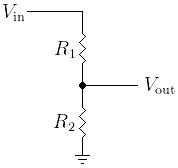# Gzalo

ES · EN

## Resistive divider calculator

Given the two values of the resistors and an input voltage, this page calculates the output voltage of the resistor divider.

R1: Ohms

R2: Ohms

Vin [Volts]

VoutA resistive divider (also called voltage divider) is a linear circuit that outputs a `Vout` voltage which is a fraction of `Vin` (input voltage).

The circuit itself is based on two resistors in series, taking the middle node as the output. It’s typically used to create a reference or to attenuate a signal.

Whenever a load is connected to the output node, a loading effect can be seen (the output voltage drops). To avoid this, the current of the resistors should be higher than the load current. The disadvantage of doing this is that efficiency is low, as most of the total current dissipates as heat outside of the load.

The output resistance is the equivalent of the parallel of both resistors, `Rout = (R1*R2)/(R1+R2)`.